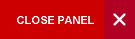## Thermodynamics and Kinetics of Materials

Semester 2

This course introduces students to the thermodynamics and kinetics of materials.  The laws of thermodynamics are reviewed along with concepts of kinetics and free energy in solid state physics.  These concepts are then used to define equilibrium.  Kinetics and equilibrium thermodynamics are combined to explain activated processes, phase diagrams, nucleation and growth, and microstructural formation. The differences in the energetics of the bulk versus the surface of materials are then discussed and used to explain the performance of nanoscaled materials (nanomaterials).  An exploration of surface behavior naturally leads to the investigation of the physics of interfaces which finds application in many areas such as the performance of composites.

Syllabus:
• Review of Zeroth First, Second and Third laws of thermodynamics;
• The concept of time dependent processes and implications; examples of kinetic processes
• Gibb’s free energy; enthalpy, entropy, equilibrium, mass action expressions
• Phase equilibria; unary and binary phase diagrams; Gibbs Phase Rule; Lever Rule
• Development of microstructure; Binary Eutectic Systems; Ceramic systems
• Kinetics of phase transformations; the Avrami Equation; Ostwald ripening (coarsening), thermodynamics of curved surfaces (capillarity).
• The surface state; Energetics of the surface; Bulk versus surface properties; Nanomaterials (surface-dominated materials).
• Solid-solid interfaces; Solid-liquid interfaces; Solid-gas interfaces and the Nernst Equation; Wetting; Hydrophilic and hydrophobic materials; Composites (interface-dominated materials), e.g., asphalt, concrete, fiberglass.
Evaluation:

The course assessment will be as follows:

• One 2-hour final written examination                         60%
• One 1-hour in-course test                                            20%
• Two graded tutorials (equally weighted)                    10%
Learning Objectives:

On successful completion of this course, students should be able to:

• use kinetics, free energy and the laws of thermodynamics to define equilibrium
• calculate the free energy associated with various reactions and processes
• use phase diagrams to determine the constitution of binary alloys and compounds
• evaluate the time-dependent constitution of solid state transformations
• evaluate the effect of curvature on thermodynamic driving forces
• examine and evaluate microstructural evolution
• distinguish between the energetics of the surface of a material and the bulk of a material
• use Nernst’s Equation to calculate the electrical potential difference between electrodes at different chemical potentials
• use the physics of interfaces to analyze the performance of composites
PHYS2561

Prescribed

• Callister, W. D. and Rethwisch D. G. (2009), Materials Science and Engineering: An Introduction, Wiley, 8th Edition. ISBN-10: 0470419970; ISBN-13: 978-0470419977

Highly Recommended

• R. T. Dehoff, R.T. (2006), Thermodynamics in Materials Science, CRC Press, 2nd Edition. ISBN-10: 0849340659; ISBN-13: 978-0849340659

Online Resources:

Course Code:
PHYS3565
Credits:
3 Credits
Level:
Level 3
Top of Page